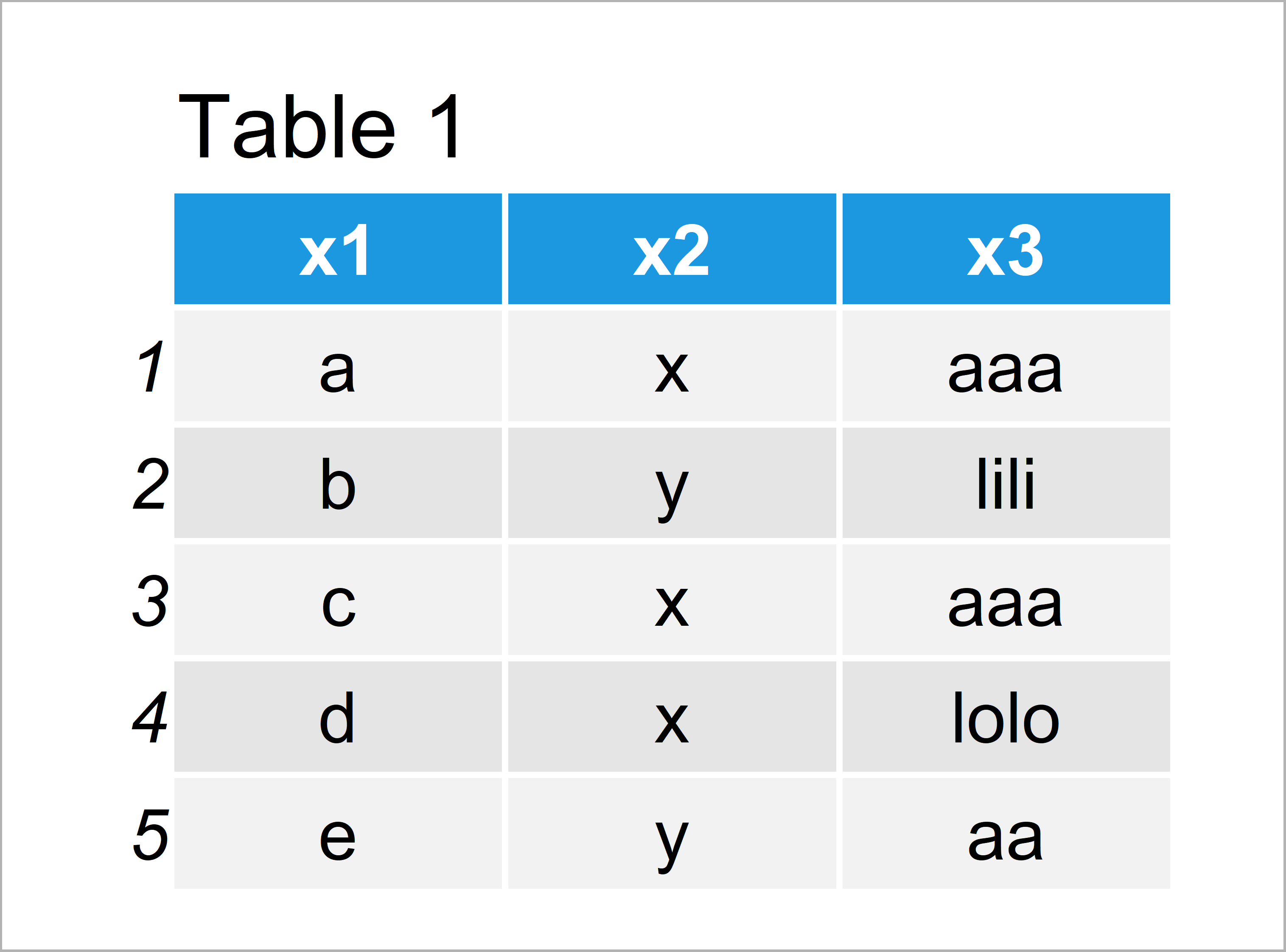# Get All Factor Levels of Vector & Data Frame Column in R (2 Examples)

In this article you’ll learn how to return all levels of a factor object in the R programming language.

It’s time to dive into the examples!

## Example 1: List All Factor Levels of Vector

The following code shows how to print all levels of a factor vector to the RStudio console.

First, we have to create an exemplifying vector in R:

```vec <- factor(c("A", "x", "bb", "A", "bb", "Hey"))  # Create example vector
vec                                                 # Print example vector
#  A   x   bb  A   bb  Hey
# Levels: A bb Hey x```

Our example vector contains six vector elements and several different factor levels.

We can now apply the levels function to return all unique factor levels of our vector:

```levels(vec)                                         # Return all factor levels of vector
#  "A"   "bb"  "Hey" "x"```

The previous output of the RStudio console shows the four different levels of our example vector.

## Example 2: List All Factor Levels of Data Frame Columns

In this example, I’ll explain how to determine the factor levels contained in a data frame.

First, we have to create a data frame in R:

```data <- data.frame(x1 = factor(letters[1:5]),       # Create example data
x2 = factor(c("x", "y", "x", "x", "y")),
x3 = factor(c("aaa", "lili", "aaa", "lolo", "aa")))
data                                                # Print example data```In Table 1 you can see that we have managed to create a data frame with three factor variables.

We can now apply the levels function to only one column to get the factor levels of this specific column:

```levels(data\$x1)                                     # Return all factor levels of column
#  "a" "b" "c" "d" "e"```

If we want to know the factor levels of each column of our data frame, we can apply the levels function in combination with the sapply function:

```sapply(data, levels)                                # Return all factor levels of each column
# \$x1
#  "a" "b" "c" "d" "e"
#
# \$x2
#  "x" "y"
#
# \$x3
#  "aa"   "aaa"  "lili" "lolo"
#```

As you can see, we have created a list where each list element contains the factor levels of one of our data frame columns.

## Video & Further Resources

Do you need more explanations on the R programming syntax of this article? Then you may want to have a look at the following video of my YouTube channel. In the video, I explain the contents of the present tutorial:

Furthermore, you might have a look at the other tutorials of my homepage. I have released numerous articles on topics such as ggplot2, factors, and graphics in R.

To summarize: In this article, I have illustrated how to return all factor levels of a vector or data frame in the R programming language. Don’t hesitate to let me know in the comments section, in case you have any further questions.

Subscribe to the Statistics Globe Newsletter